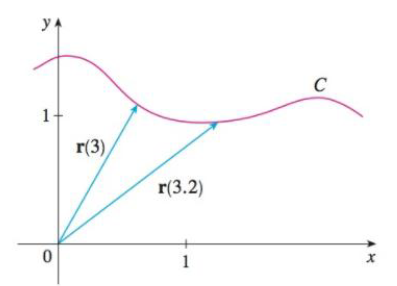Chapter 13, Problem 16RE

Chapter
Section
Textbook Problem

# The figure shows the curve C traced by a particle with position vector r(t) at time t.(a) Draw a vector that represents the average velocity of the particle over the time interval 3≤ t ≤ 3.2.(b) Write an expression for the velocity v(3).(c) Write an expression for the unit tangent vector T(3) and draw it.(a)

To determine

To draw: A vector that represents the average velocity of the particle over the time interval 3t3.2.

Explanation

Given data:

The figure shows the curve C traced by the particle with position vector r(t) at time t.

The interval of the time is given as 3t3.2.

Formula used:

The expression to find the average velocity of the particle over the time interval atb is,

ravg-velocity=r(b)r(a)ba (1)

Calculation:

Calculation of average velocity vector is as follows.

Substitute 3.2 for b and 3 for a in equation (1),

ravg-velocity=r(3.2)r(3)3.23=r(3.2)r(3)0.2=5[r(3.2)r(3)]

Therefore, the average velocity vector of the particle over the time interval 3t3

(b)

To determine

To find: The expression for the velocity v(3).

(c)

To determine

To write: The expression for the unit tangent vector T(3).

### Still sussing out bartleby?

Check out a sample textbook solution.

See a sample solution

#### The Solution to Your Study Problems

Bartleby provides explanations to thousands of textbook problems written by our experts, many with advanced degrees!

Get Started

#### Solve the equations in Exercises 126. 2x3x2x3x+1=0

Finite Mathematics and Applied Calculus (MindTap Course List)

#### Find a function f and a number a such that limh0(2+h)664h=f(a)

Single Variable Calculus: Early Transcendentals, Volume I

#### Evaluate the integral. 3. 14ylnydy

Single Variable Calculus: Early Transcendentals

#### Let A=B=C= Find a AB, b (A+CT)B, and c CTBABT.

Finite Mathematics for the Managerial, Life, and Social Sciences

#### Using tan2 x = sec2 x − 1, ∫ tan3 x dx =

Study Guide for Stewart's Single Variable Calculus: Early Transcendentals, 8th